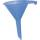# Cube + third power - math problems

#### Number of problems found: 85

• Rectangle poolFind dimensions of an open pool with a square bottom with a capacity of 32 m3 to have painted/bricked walls with the least amount of material.
• CalculateCalculate the surface of the cube if its volume is 64 cm ^ 3.
• Cube diagonalsCalculate the length of the side and the diagonals of the cube with a volume of 27 cm3.
• FunnelThe funnel has the shape of an equilateral cone. Calculate the surface wetted with water if we poured into the funnel 7.1 liters of water.
• Cube 7Calculate the volume of a cube, whose sum of the lengths of all edges is 276 cm.

We apologize, but in this category are not a lot of examples.
Do you have an exciting math question or word problem that you can't solve? Ask a question or post a math problem, and we can try to solve it.

We will send a solution to your e-mail address. Solved examples are also published here. Please enter the e-mail correctly and check whether you don't have a full mailbox.

Cube Problems. Third power - math problems.